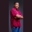Related Tags

swift
communitycreator

# What is array.max() in Swift?Theodore Kelechukwu Onyejiaku

### Overview

The array.max() is used to get the largest element in an array.

### Syntax

arr.max()


### Parameters

The function takes no parameters.

### Return value

It returns the largest element in the array. If the array has no elements, a nil is returned.

### Example

Let’s look at the code below:

// creating arrays
let evenNumbers = [2, 10, 4, 12, 6, 8]
let negativeNumbers = [-1.3, -0.5, 0.002, 4.5]
let decimalNumbers = [45.7, 44.2, 90.0, 23.3]
let emptyArray = [Int]() //empty array

// getting maximum elements
let maxEvenNo = evenNumbers.max()!
let maxNegNo = negativeNumbers.max()!
let maxDecNo = decimalNumbers.max()!
let emptyElement = emptyArray.max()

// printing results
print(maxEvenNo)  // 12
print(maxNegNo)   // 4.5
print(maxDecNo)   // 90.0
print(emptyElement) // nil
Using the max() method

### Explanation

• Lines 2 to 5: We create three separate arrays.
• Lines 8 to 11: We invoke the max() method on each of the arrays and the results are stored in separate variables.
• Lines 14 to 17: We print the results to the console.

Note: For an empty array, the max() function returns a nil and throws an error.

### max(by:)

max(by:) method is similar to the max() method. The only difference is that it finds the maximum element based on a predicate or a condition.

### Example

Let’s look at the code example:

// create a sequence
let ages = ["Mark" : 15, "John" : 20, "Jane" : 40]

// get maximum element using max(by:)
let keyMaxElement = ages.max(by: {
$0 >$1
})

// print result
print(keyMaxElement!)
Using the max(by:) method

## Explanation

• Line 2: We create a sequence with the name ages.

• Lines 6 to 7: We write a predicate to get the maximum element greater than all other elements. Here, the greatest key value is 40.

• Line 11: The result is printed.

RELATED TAGS

swift
communitycreator

CONTRIBUTORTheodore Kelechukwu Onyejiaku
RELATED COURSES

View all Courses

Keep Exploring

Learn in-demand tech skills in half the time